Equilibrium (2020 VCE) 1) The magnitude of the equilibrium constant, Kc, at 25 °C for the following reaction is 640.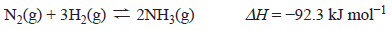For the reaction, below, the magnitude of Kc at 25 °C is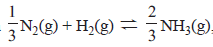A. 9 and ΔH = −30.8 kJ mol- B. 213 and ΔH = −30.8 kJ mol- C. 640 and ΔH = −30.8 kJ mol- D. 640 and ΔH = −92.3 kJ mol- Solution Question 2 For the reaction below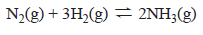A. a catalyst increases the number of collisions between the reactants. B. the rate of the forward reaction increases when the temperature increases. C. a catalyst reduces the activation energy of the forward and backward reactions by the same proportion. D. the activation energy of the forward reaction is greater than the activation energy of the reverse reaction Solution3) The following equation represents the reaction between chlorine gas, Cl2, and carbon monoxide gas, CO.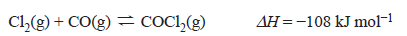The concentration–time graph below represents changes to the system.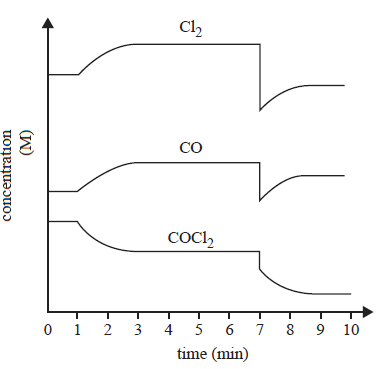Which of the following identifies the changes to the system that took place at 1 minute and at 7 minutes?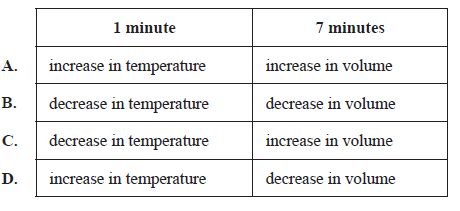Solution3) Nitrogen dioxide, NO2, and dinitrogen tetroxide, N2O4 equation.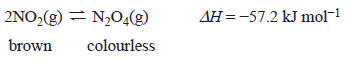A change was made at time t1 to an equilibrium mixture of NO2 and N2O4, which achieved a new equilibrium at time t2. A graph showing the rate of the forward reaction is shown below.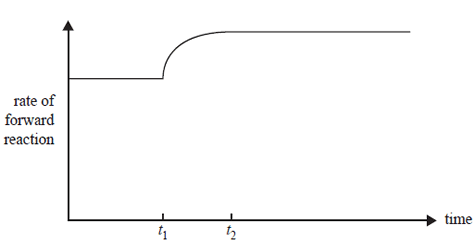Which one of the following describes the change that was made to the initial equilibrium system and the colour change that occurred between t1 and t2? A. The temperature was increased and the colour lightened. B. The temperature was increased and the colour darkened. C. The temperature was decreased and the colour lightened. D. The temperature was decreased and the colour darkened. Solution4) Methanol is a very useful fuel. It can be manufactured from biogas. The main reaction in methanol production from biogas is represented by the following equation.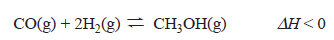This reaction requires the use of a catalyst to maximise the yield of methanol produced in optimum conditions. The energy profile diagram below represents the uncatalysed reaction.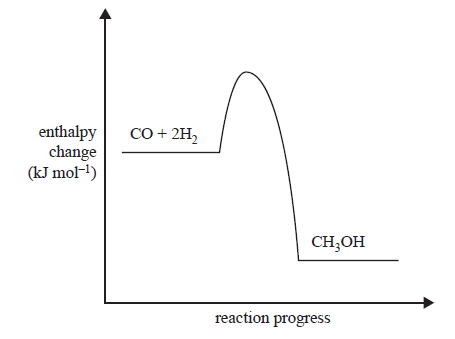i. How does the reaction temperature affect the yield of methanol from biogas? In your answer, refer to Le Chatelier’s principle. Solution ii. How does the reaction pressure affect the yield of methanol from biogas? In your answer, refer to Le Chatelier’s principle. Solution iii. Write the expression for the equilibrium constant, Kc, for this reaction. Solution iv. 0.760 mol of carbon monoxide, CO, and 0.525 mol of hydrogen, H2, were allowed to reachequilibrium in a 500 mL container. At equilibrium the mixture contained 0.122 mol of methanol. Calculate the equilibrium constant, Kc. Solution